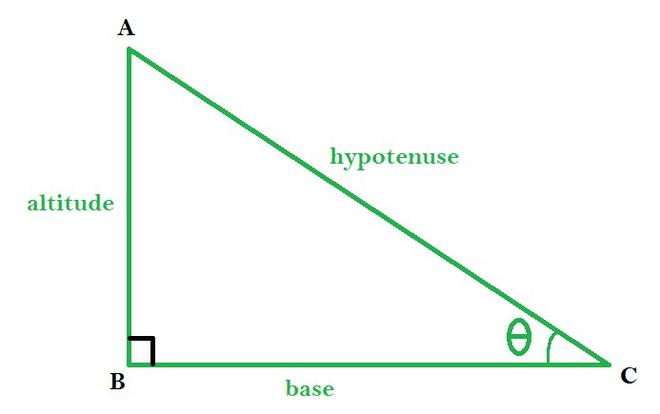# How do I get the angle between two parts?

Hello everyone,

So I was wondering how to get the angle between two parts on a flat surface. Thank you!

1 Like

Well, something like this: Best way to get the angle between two vectors? - #4 by ThanksRoBama

Although “the angle between two parts” doesn’t really make sense. You need 3 points or 2 vectors to talk about angles.

1 Like

I mean like, I need theta. Thanks for your answer. There are three points, its just A = Part1, B = Right Angle, C = Part 2. So I need the angle between B and C if that helps.

1 Like

Dot Product might work for you

Dot product gives you a value from -1 to 1. Both 1 and -1 means the vectors are parallel, while 0 means the vectors are perpendicular.

Then you multiplayer that value by 360 for degrees, or 2pi for radians.

The code below demonstrates this (C would be the position of the right angle)

``````local corner = C.Position
local aDir = (A.Position - corner).Unit
local bDir = (B.Position - corner).Unit

local angleDeg = aDir:Dot(bDir) * 360

``````

Okay, I will try this, thanks. But how do I get the points A, B, and C in the first place again? Like from the two parts.

1 Like

Please post a diagram or a screenshot or something

3 Likes

What I mean is like, there are two straight lines and a 90 degrees that joins together two points on a plane. I know how to get the angle on a 2D surface just not on a 3D one.

EDIT: Here is a diagram, thanks guys. C is one part, A is another part.I’m had this problem for a long time too, i’m used this function to fix my problem and make this cool turret

``````local x1,y1,z1 = CFrame.lookAt(base.Position,mouse.Hit.p):ToObjectSpace(base.CFrame):ToOrientation()
``````

or use math.atan2 to get the angle beetween difference X and difference Z of two parts and remember to multiply it by (180/pi)

1 Like

it will give you a angle relative to another parts look vector

Thanks, but i’m not trying to deal with anything with a mouse. I just need the 3D angle between one part and another. Kind of like how you solve a right angled triangle.

1 Like

it’s an example, you can put there part1 and part2 positions instead
or do that

``````
local x, y, z = CFrame.LookAt(Pos1, Pos2):ToWorldSpace():ToOrientation()

local AngleThatYouCanFindExample = y * (180/math.pi)

-- do code
``````

This doesn’t really make sense since there is no real way to just ‘get angles’ between two parts.

Do you by chance mean that you want help calculating the difference between two angles? That is possible if you are asking.

1 Like

it’s not possible , but if you project vector from part 1 to part 2 you will get the third point and then make atan2 to do this or if you wan’t to project it without math use my function above

1 Like